Jekyll2023-03-12T21:24:39+00:00https://shubham1810.github.io/feed.xmlblankMy Personal Webpage Displaying External Posts on Your al-folio Blog2022-04-23T23:20:09+00:002022-04-23T23:20:09+00:00https://shubham1810.github.io/blog/2022/displaying-external-posts-on-your-al-folio-bloga post with redirect2021-07-04T17:39:00+00:002021-07-04T17:39:00+00:00https://shubham1810.github.io/blog/2021/redirectSemi-Implicit Networks2019-07-19T11:00:00+00:002019-07-19T11:00:00+00:00https://shubham1810.github.io/blog/2019/imexResidual Neural Networks, or ResNets , became popularised in the recent years, Making it possible to train very deep neural networks while still achieving compelling performance. The core idea behind ResNets is the addition of skip-connections which enables the network to avoid the problem of vanishing gradients upto a large extent, and hence, making it easier for the network to be very deep (One of the examples is ResNet’s 152 layers, compared to VGG’s 19 layers or GoogleNet’s 22 layers ).

The similarity of ResNet architecture with Ordinary Differential Equations has been under some attention in recent works . The connection raises the issue of forward stability of such methods i.e. the model should not amlify the features through layers when perturbations such as noise, adversarial attacks or general changes appear in the given input.

This post closely follows (read shamelessly copies) the work presented in IMEXnet - Forward Stable Deep Neural Network (Published in ICML 2019). In this work, Authors talk about the forward stability of residual architectures and the problems that can arise with using explicit methods for the ordinary differential equation forms of ResNet. The authors also look closely at the field of view problem in terms of high-dimensional output problems (such as image-to-image methods like segmentation, depth-estimation, super-resolution etc.). For solving tasks that involve high-dimensional output, several layers of Residual blocks are often employed in the network architecture to model interactions between far away pixels. The authors introduce an architecture based on Implicit-Explicit methods for the ODE/PDE form of the Residual Networks which enhances the field of view with an improvement in stability of the network.

In this post, I have discussed the concept of semi-implicit methods provided by the authors. For a detailed view and experimental analysis, head over to their paper and their github repo

## Residual Method as ODE

The $j^{th}$ layer of a Residual layer, updating the feature $Y_j$ can be written as:

\begin{equation} Y_{j+1} = Y_{j} + h.f(Y_{j}, \theta_{j}) \tag{1} \label{eq:one} \end{equation}

Where, $Y_{j+1}$ and $Y_j$ are outputs of layers $j+1$ and $j$ respectively. $\theta_j$ is the layer parameter, $f$ is a non-linear function, and $h$ is the step size (usually set to 1). In problems related to images, the function $f$ is usually a series of convolutions, normalisation and activations. In this particular work, $f$ is taken to be:

\begin{equation} f(Y, K_1, K_2, \alpha, \beta) = K_2 \sigma (N_{\alpha,\beta} (K_1 Y)) \tag{2} \label{eq:two} \end{equation}

Here $K_1$ and $K_2$ are taken to be 3x3 convolutional kernels, $N_{\alpha,\beta}$ is the normalization layer and $\sigma$ is the non-linear activation function. This structure was taken from . The function in the above equation can be used to see that the operation on a small 5x5 patch will be used to evaluate the output pixel information, thus making it necessary to use a number of such blocks to have a wider field of view over the input image.

### Forward Euler Form

In lieu of the step function described in \eqref{eq:one} (the discretized form), the forward euler formulation of the ODE is written as:

\begin{equation} \dot Y(t) = f(Y(t), \theta(t))
Y(0) = Y_0 \tag{3} \label{eq:three} \end{equation}

The features $Y(t)$ and the weights $\theta(t)$ are taken to be continuous functions in time, where $t$ corresponds to the depth of the network. Previously, explicit methods (such as mid-point method, Runge Kutta method) have been utilised to solve such equations, they often suffer from a lack of stability. Explicit methods are of the form where the information in $Y_{t+1}$ is described as a functoin of the previous state $Y_t$. Using some iterative methods (as mentioned in examples above), many small steps are usually needed to integrate the PDE over a long amount of time.

As mentioned in the paper, one way to improve the flow of information in the network modelled after ODEs is to make use of implicit methods, i.e. express the state $Y_{t+1}$ in terms of the same time-step $Y_{t+1}$ implicitly.

## Semi-Implicit Form and It’s Stability

One of the simplest forms for implicit functions, quite similar to forward euler equation is the backward euler method in the non-linear discretized form:

\begin{equation} Y_{j+1} - Y_{j} = h . f(Y_{j+1}, \theta_{j+1}) \tag{4} \label{eq:four} \end{equation}

This method is stable for any choice of $h$ when the eigenvalue of the jacobian of $f$ have no positive real part (See This article for more details on stability of methods w.r.t to second-order differential equations). If the given condition is satisfied, $h$ can be chosen large enough to simulate large step-size in the continuous form while being robust to small perturbations in the input information.

Turns out, implicit methods are rather expensive to compute. Especially the above mentioned equation \eqref{eq:four} is a non-linear problem which can be computationally expensive to solve. So rather than using a full implicit or explicit method, the authors derived a combination in the form of a implicit-explicit (IMEX) or semi-implicit method.

They key idea in IMEX methods is to divide the right-hand side of the ODE into two parts: A non-linear explicit form and a linear implicit form. The equation in IMEXnet is designed in such a way that it can be solved efficiently. The equation in \eqref{eq:three} will now be reformatted as:

\begin{equation} \dot Y(t) = f(Y(t), \theta(t)) + LY(t) - LY(t) \tag{5} \label{eq:five} \end{equation}

where, The first part $f(Y(t), \theta(t)) + LY(t)$ is treated explicitly, while the second part $LY(t)$ is treated implicitly.
The matrix $L$ is chosen freely with the property of being easily invertible. A fair choice of $L$ can be modelled after a 3x3 convolution operation with symmetric positive-definite property, which makes it easy to invert (more on that later). The continuous equation can now be simplified as the following:

$Y_{j+1} - hLY_{j+1} = Y_j + hf(Y_j, \theta_j) + hLY_j$

which can be simplified as:

\begin{equation} Y_{j+1} = (I - hL)^{-1} (Y_j + hLY_j + hf(Y_j, \theta_j)) \tag{6} \label{eq:six} \end{equation}

with $I$ being the identity matrix.
In the above equation, the authors have shown that the forward part (while seemingly complex) is rather easy to compute and similar to that of a convolution. Furthermore, the authors claim that the network is always stable for a suitable choice of $L$, while having some favourable properties of implicit methods. The matrix $(I + hL)^{-1}$ is dense in nature, which avoids the field of view problem by using all pixels of the image in it’s computational step.

The authors choose $L$ to be a laplacian matrix with a group convolution operator (group conv. was also used in AlexNet! . The weights of the matrix are taken as the following:

$\begin{equation} L = \frac{1}{6} \begin{bmatrix} -1 & -4 & -1 &\\ -4 & 20 & -4\\ -1 & -4 & -1 \end{bmatrix} \tag{7} \end{equation}$

Before going into the discussion about the choice of $L$ and the stability of the method, a quick recap of the Laplace transform is due.

The [Laplace transform](https://en.wikipedia.org/wiki/Laplace_transform) (taken from wikipedia), converts a function of real variable $t$ to a function of a complex variable $s$. The laplace transform for $f(t); t \ge 0$ is the function $F(s)$ which is a unilteral transform defined by: $$F(s) = \int_{0}^{\infty} f(t) e^{-st} dt$$ And, for a laplacian matrix, $L$ is defined as, $L = D - A$ for a graph $G$, where $A$ is the adjacency matrix and $D$ is the degree matrix of the graph $G$.

Now, on the stability of the method, the authors provide a wonderful example of a simplified setting with a model problem (as given below) and provide the reasoning for the aforementioned choice of $L$.

\begin{equation} \dot Y(t) = \lambda Y(t)
Y(t) = Y_0 \tag{8} \end{equation}

And take $L = \alpha I$, where we choose $\alpha \ge 0$. (Refer to the paper for a complete proof). Based on the analysis, the authors choose $K_1 = -K_{2}^{\intercal}$ in the equation \eqref{eq:two} as discussed properly in , and also impose bound constraints on the convolution weights to achieve a bound on the term of $\lambda$, hence improving the stability of the model.

An example of the field of view is shown here for IMEXnet.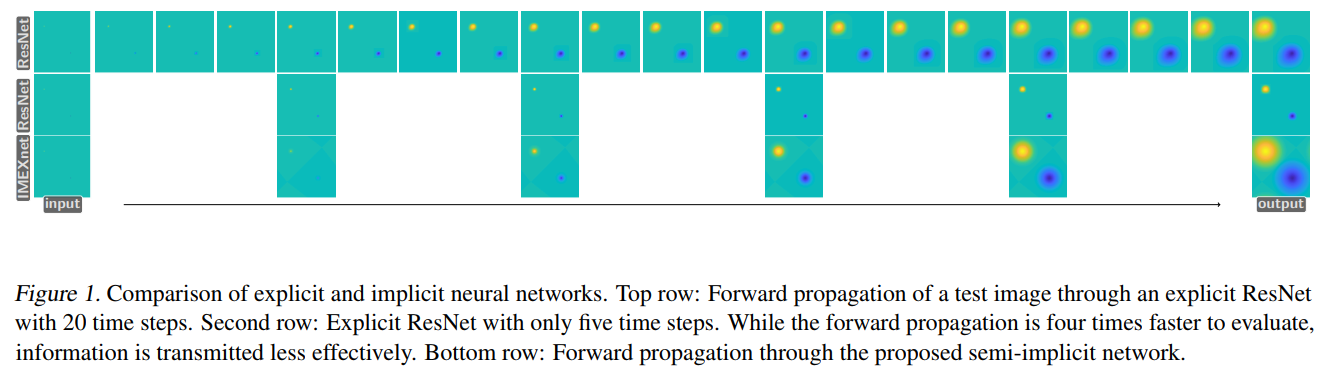### The Forward Pass

The authors show that using already available and widely used tools such as auto-differentiation and the fast fourier transform (FFT), an efficient way for computing the linear system given below can be found.

$(I + hL)Y = B$

where, $L$ is constructed like a group-wise convolution as mentioned earlier and $B$ collects the explicit term.

For efficient solution to the system, authors make use of the convolution theorem in the fourier space. The theorem says, for a convolution operation between a kernel $A$ and features $Y$, the convolutional operation can be computed as:

\begin{equation} A * Y = F^{-1}((FA) \odot (FY)) \tag{9} \label{eq:nine} \end{equation}

Where, $F$ is the Fourier transform, $*$ is the convolution operator, and $\odot$ is the hadamard-product (element-wise multiplication). Here, we assume a periodic boundary on the image data (discussed in detail next). This implies that if we need to compute the product of inverse of the convolutional operator $A$, we can simply element-wise divide by the inverse fourier transform of $A$:

$A^{-1} * Y = F^{-1}((FY) \oslash (FA))$

In our case, the kernel $A$ is associated with the matrix $I + hL$, which is invertible. For example, when we choose $L$ to be positive semi-definite, we define:

$L = B^{\intercal} B$

Where, $B$ is a trainable group-convolution operator. Using Fourier methods, we need to have the convolutional kernel at the same size as the image we convolve it with. This is done by generating a zero-matrix as the same size as that of the image and inserting entries of the kernel at appropriate places.

For a more thorough explaination about how to construct this kernel for fourier method, refer to the book . The periodic boundary condition and the positive semi-definite property of the kernel are important here to derive the final convolution kernel $A$ for fourier transform and it’s spectral decomposition. Specifically, in chapters 3 and 4 of the book, it is given in detail about how to form the convolution kernel (or toeplitz matrix) for the __BCCB (Boundary Circulant with Circulat Blocks)__ type matrix. All BCCB matrices are normal in nature, i.e. $A^{*} A = A A^{*}$. So, a basic outline to compute the equation \eqref{eq:nine} is:
1. Compute the center of the kernel (after zero padding to match the size)
2. Apply the corresponding circular shift over the kernel with the center.
3. Compute the fourier transform of the update kernel and the image.
4. Take the inverse fourier transform of the product.
Refer to for a detailed information about the process, and [convolution theorem](https://en.wikipedia.org/wiki/Convolution_theorem)</a> for a proof of the equation \eqref{eq:nine}.

The method is wonderfully captured by the authors with the help of a PyTorch pseudo-code as following: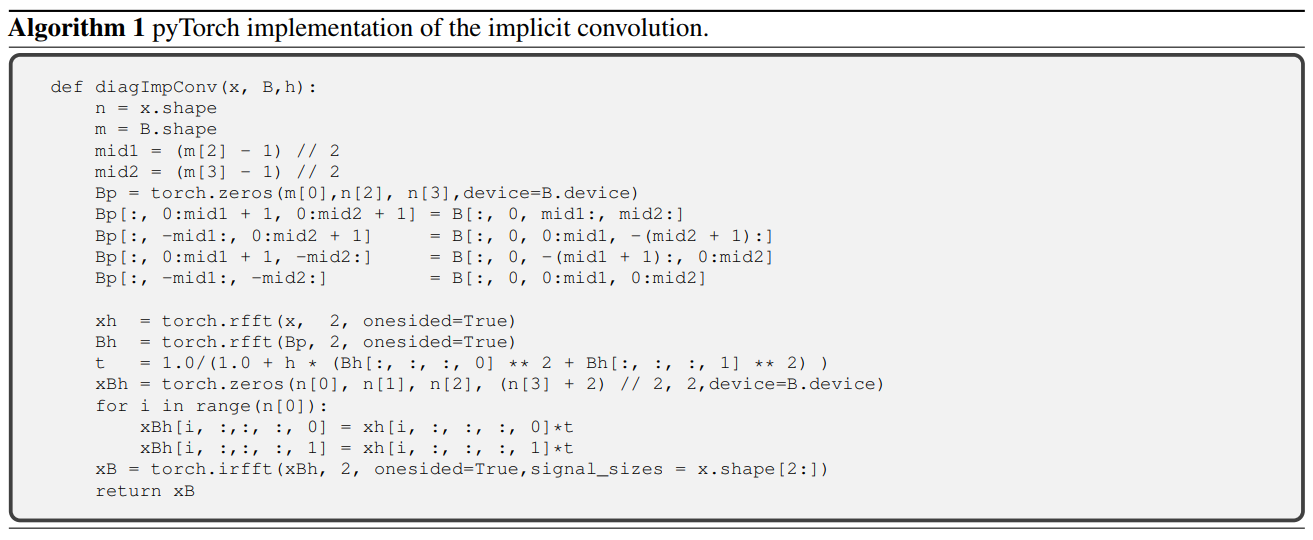### Computational Complexity

For a single block ResNet, with m channels and input image of size sxs, the forward pass takes approximately $\mathcal{O}(m^2 s^2)$ operations and $\mathcal{O}(m^2)$ memory.

For the IMEX network, the explicit is pretty much the same followed by the implicit step. The Implicit step is a group-wise convolutional operation and requires $\mathcal{O}(m(s.log(s))^2)$ additional operations. The $s.log(s)$ term results from the application of the fourier transform. Since $log(s)$ is typically much smaller than $m$, the additional cost can be considered insignificant.

## Final Notes

As for the effectiveness of the network, the authors provide some compelling results on problems such as segmentation on synthetic Q-tip images as a toy example, and depth-estimation over kitchen images from the NYU Depth V2 dataset. One example as taken from the paper is shown below:

First example from the Qtip segmentation: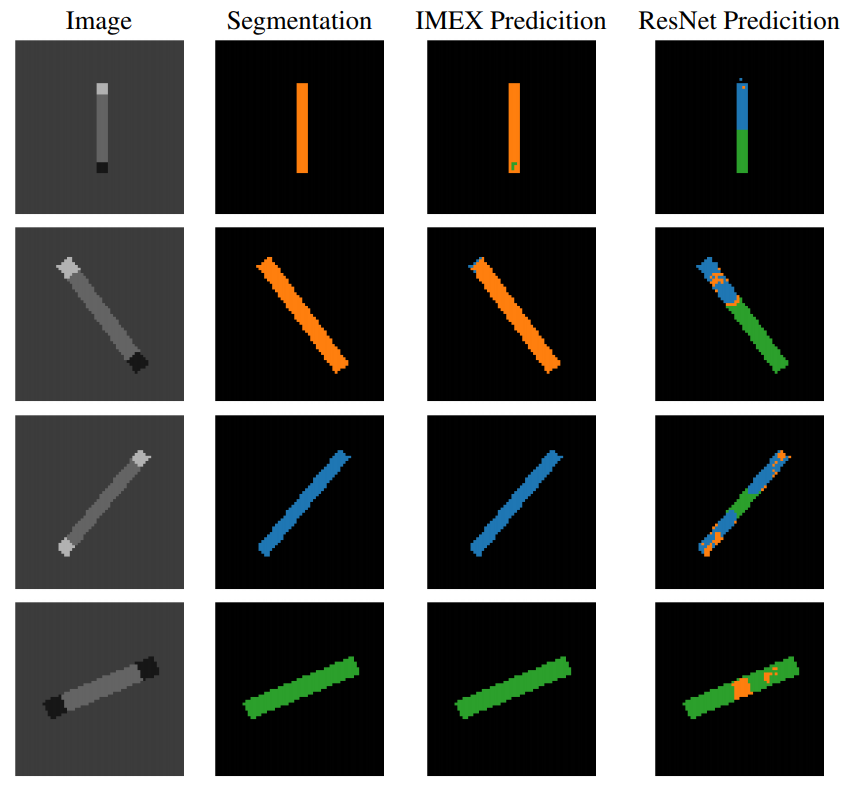And an example from the depth estimation for kitchen images taken from the NYU Depth V2 dataset .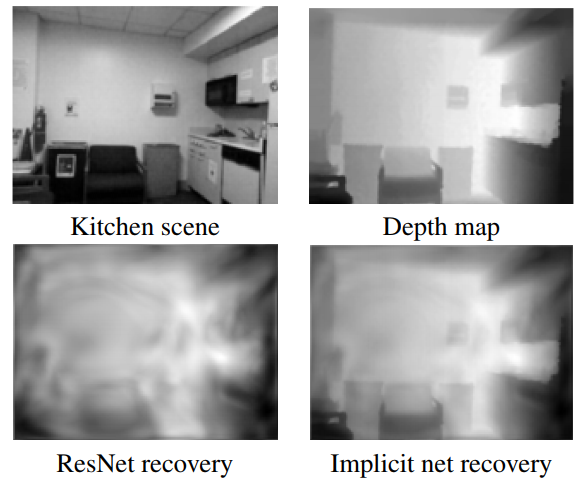The authors also make note of further possibilities for choosing other models with similar implicit properties. They epecially make note of a variant that can be used (called the diffusion-reaction problem):

$\dot Y(t) = f(Y(t), \theta(t)) - LY(t)$

Such equations can have interesting behaviour like forming non-linear wave patterns etc. These systems have been already studied in rigourous details as mentioned in the paper.

Some further work over this appproach is also discussed in the paper: Robust Learning with Implicit Residual Networks , but that is beyond the scope of this post for now.

NOTE: I have written this post as per my understanding of the paper, and for my learning. I have tried to summarize (mostly just copy) the paper to the best of my capability in a short duration. Any constructive reviews are welcome.

]]>
Shubham Dokania
Principal Component Analysis2018-06-28T09:00:00+00:002018-06-28T09:00:00+00:00https://shubham1810.github.io/blog/2018/pcaAs we work with real world data, we notice that the complexity increases; both in terms of dependency of variables on each other and dimensionality (number of variables) of the problem. Several techniques exist for analysis of such information and to make it easier to extract important properties for the purpose of better computation and visualization. One such method is the Principal Component Analysis (PCA), which emphasises on the variance of the data to extract the directions which maximize the data variation.

One of the major applications of PCA is dimensionality reduction, which is attained by choosing the transformed variables (obtained from projection of original variables on the direction of maximum variances, or the principal components).

Few of the prerequisites for understanding PCA are: Covariance, Eigenvectors, and Singular Value Decomposition.

1. Eigenvalues & Eigenvectors: Setosa visualization, 3Blue1Brown
2. SVD: This nice Medium blogpost

For example, take some data (Say, $$X$$) with zero mean (if mean is not zero then subtract all values $$x_i$$ with the mean, $$\mu$$). The covariance of this data (Say $$C_X$$) is given by:

$C_X = \frac{1}{n}\cdot X\cdot X^T$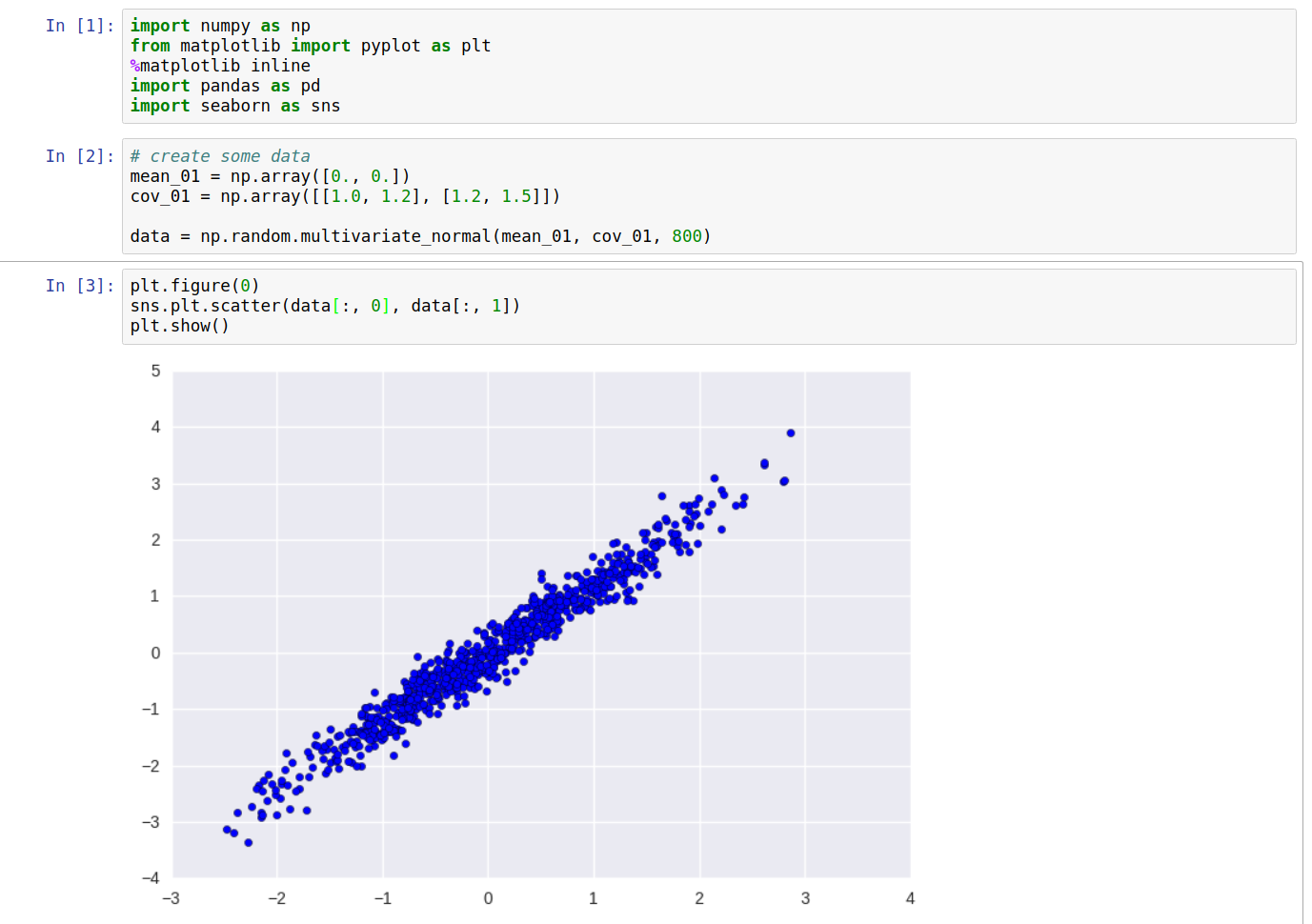We want to figure out a transformation function $$W$$ and apply on the data $$X$$ so that in the resulting data $$Y$$, the variables will be independent of each other. In simple terms, the covariance between any two distinct columns of $$Y$$ will be zero, i.e. the non-diagonal elements of the covariance matrix $$C_Y$$ of $$Y$$ will be zero. This implies that $$C_Y$$ will be a diagonal matrix.

Writing the transformation from $$X$$ to $$Y$$, we have:

$Y = X\cdot W$

To solve for the covariance matrix of Y, we can write

$C_Y = \frac{1}{n}\cdot Y\cdot Y^T$

and since, $$Y = W\cdot X$$, we have,

$C_Y = \frac{1}{n}\cdot W\cdot X\cdot (W\cdot X)^T\\ C_Y = \frac{1}{n}\cdot W\cdot X\cdot X^T\cdot W^T\\ C_Y = W\cdot (\frac{1}{n}\cdot X\cdot X^T)\cdot W^T\\ C_Y = W\cdot C_X\cdot W^T$

or,

$C_X = W^T\cdot C_Y\cdot W$

We know that, $$C_Y$$ is supposed to be a diagonal matrix. What does this equation remind us of? but of course, the Singular Value Decomposition (SVD). Thus, If we take $$W$$ as the matrix of the eigenvectors and $$C_Y$$ as the diagonal matrix of the eigenvalues, the above equation will hold true, making the matrix $$W$$, of eigenvectors of covariance of $$X$$, our transformation matrix.

Computing the above values for our data, and plotting the directions of the obtained eigenvalues, we get the following: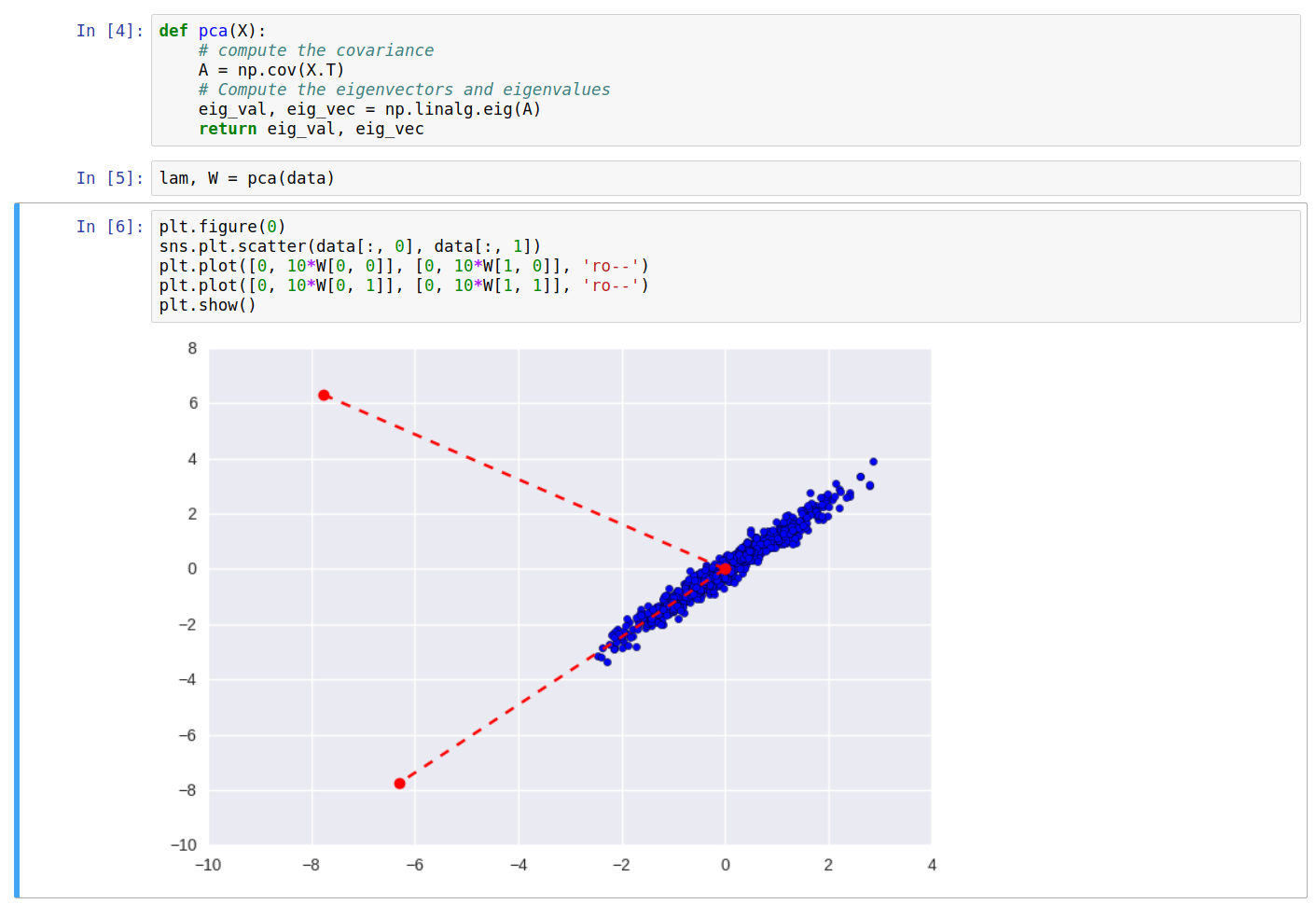As can be seen clearly, one of the eigenvectors falls along the direction of maximum variance of the data. On transforming the data $$X$$ into $$Y$$, and plotting again, we get: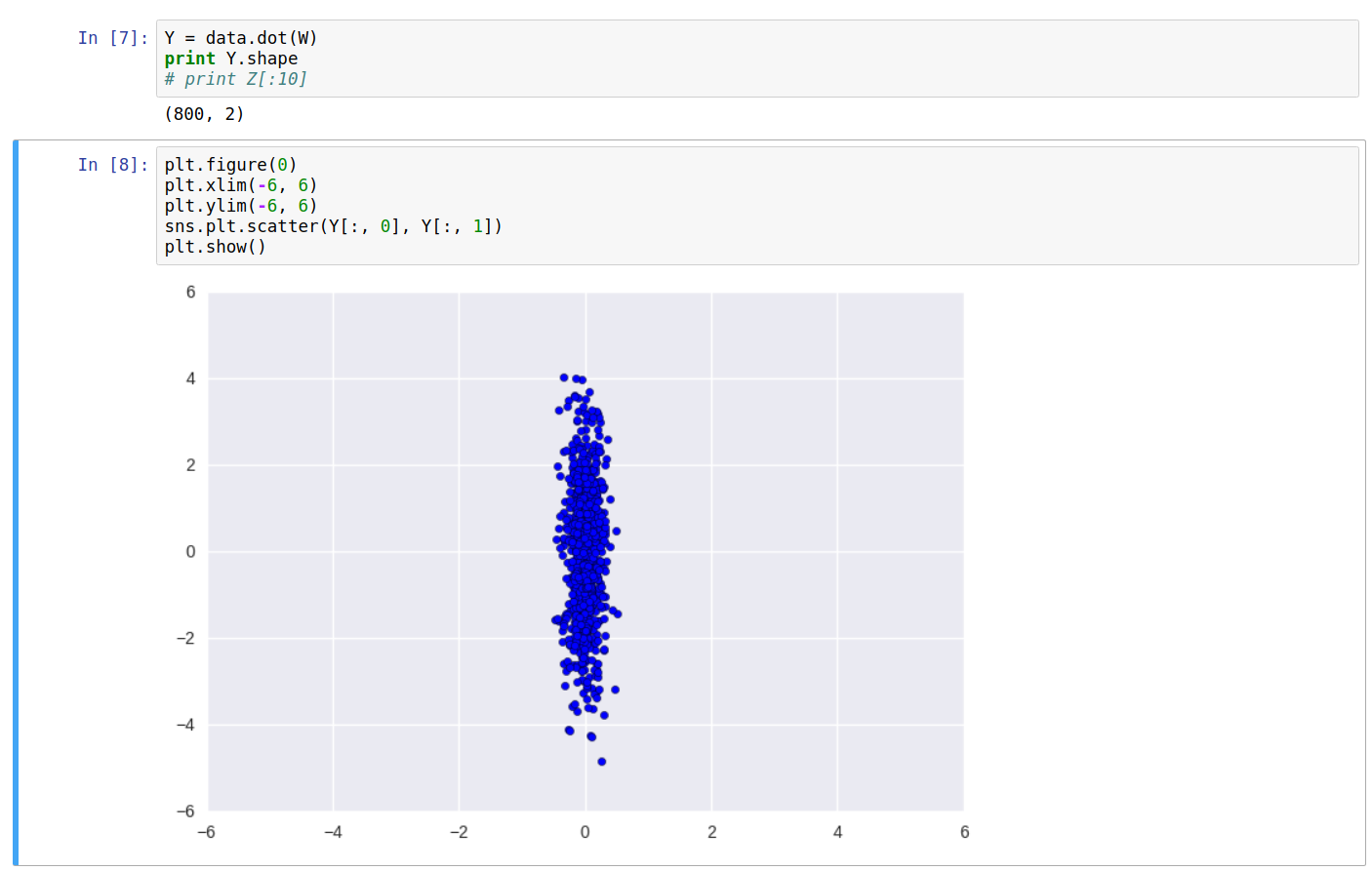Printing the covariance of the new data $$Y$$, we can see it’s a diagonal matrix. Also, the equation $$W\cdot C_Y\cdot W^T$$ returns the original covariance matrix $$C_X$$.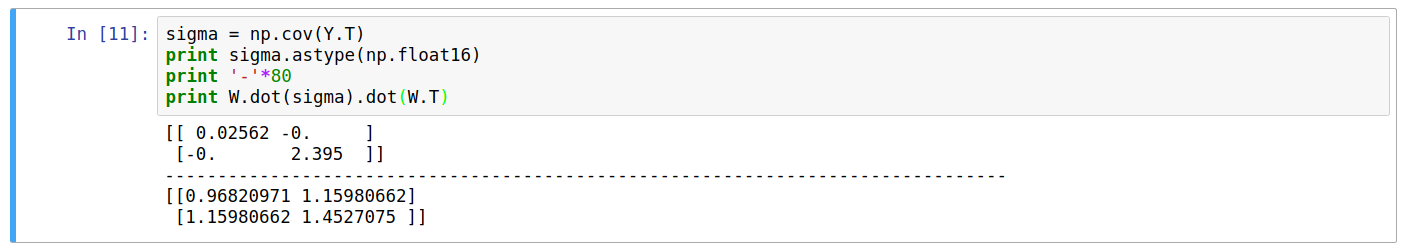### Dimension Reduction

One of the major applications of PCA is it’s ability to choose the dimensions of maximum variation, i.e. taking the projection of the data along those components only will not affect the complexity of the data by a significant amount and data can be reconstructed back to an approximation of it’s original form with the lower dimensional data as well.

On paying more attention to the covariance matrix $$C_Y$$, we see that the magnitude of the eigenvalues along the diagonal of the matrix is related to the amount of variances explained by the said eigenvector direction.

So, sorting the eigenvalues and corresponding eigenvector pairs in decreasing order and taking only the top values becomes the ideal way of choosing the eigenvectors for obtaining maximum explained variances.

For further demonstration, let’s use another dataset (MNIST) for PCA.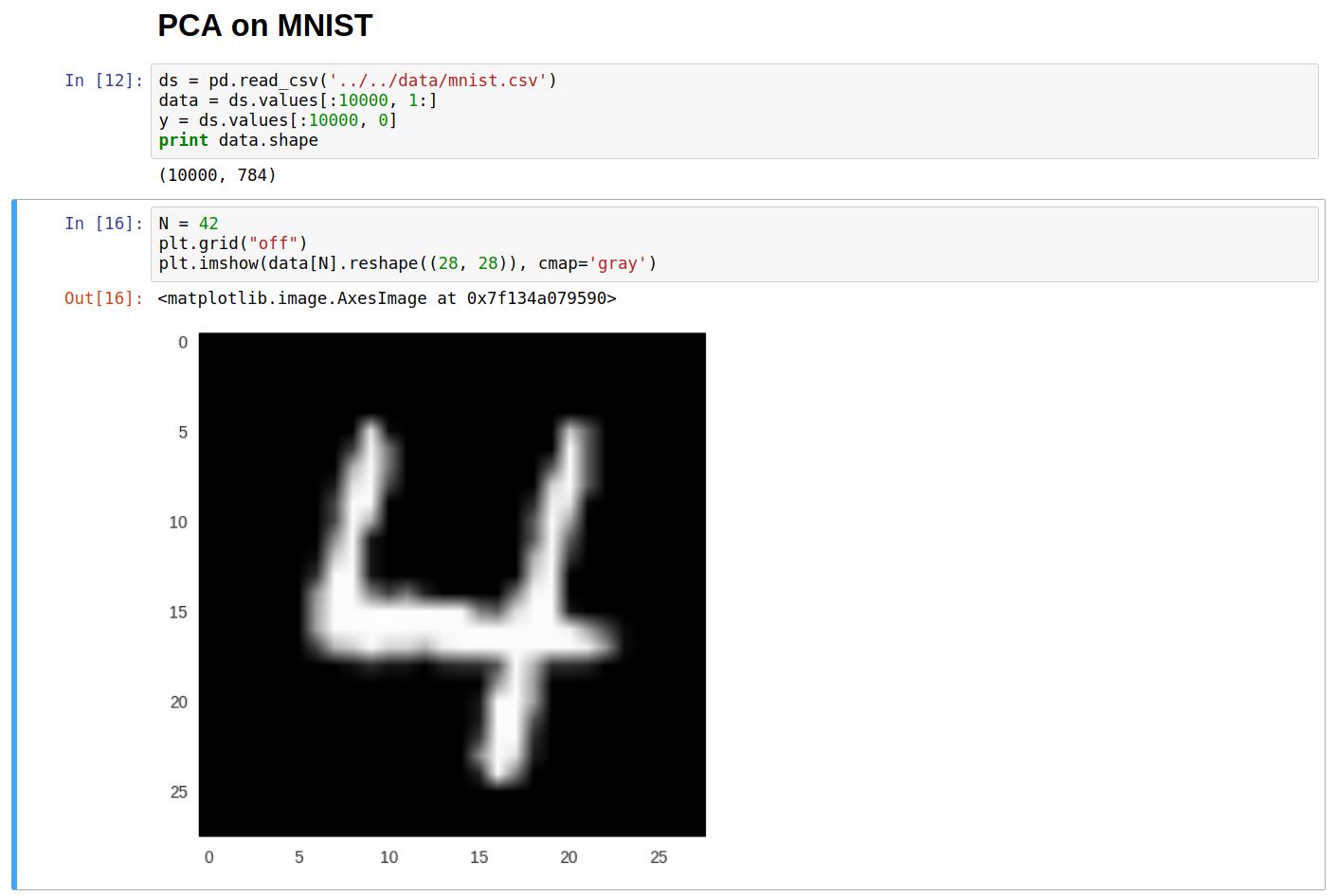Computing the eigenvectors and eigenvalues for the above dataset and sorting them on the basis of eigenvalues (descending order), we can store them back in numpy arrays.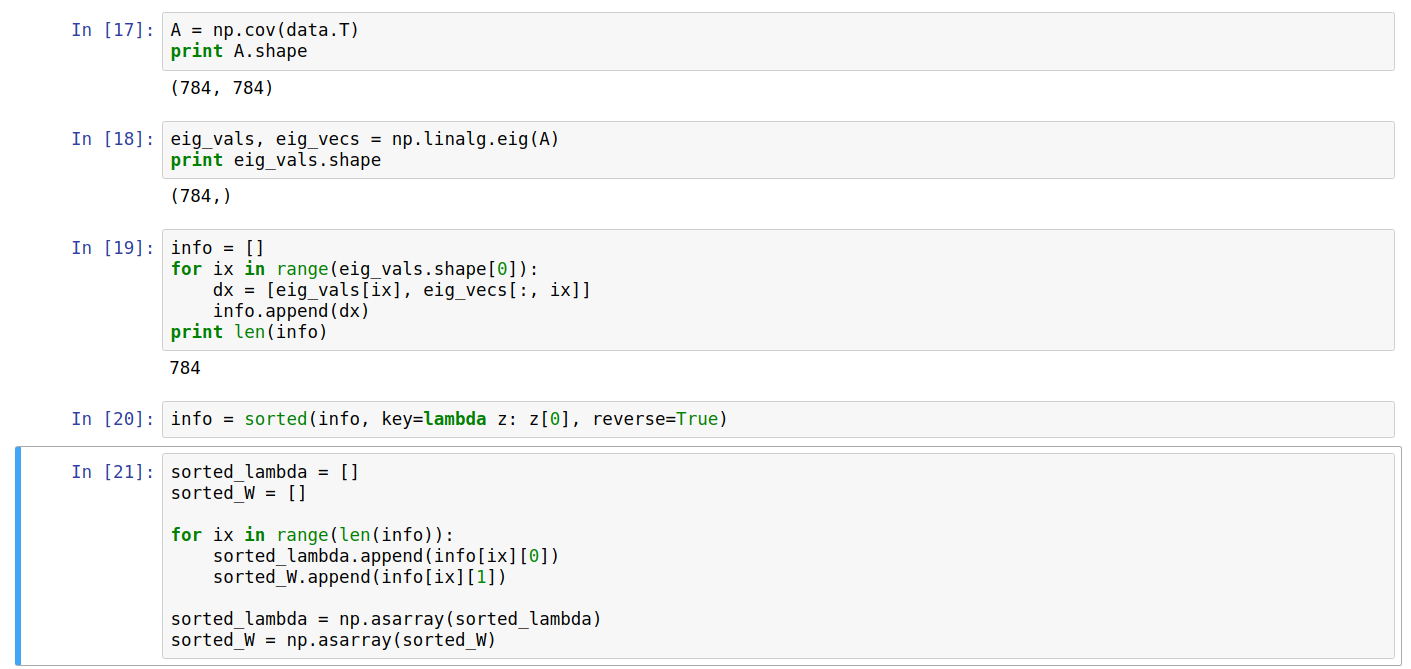And plot the eigenvalues, and the cumulative sum of the eigenvalues (Explained Variances).From the above curve for the cumulative sum, denoting the explained variances of the original data, we can conclude that approximate 150 dimensions shall be enough to get ~95% of the variances of the original dataset, and about 326 dimensions out of 784 for ~99%.

To reduce the number of dimensions, we have to select the number of dimensions we want $$k$$ and use only those $$k$$ columns from $$W$$ to form the transformation matrix (Say $$W'$$). Thus the transformation and reconstruction operation become:

$Y_{m \times k} = X_{m \times n} \cdot W'_{n \times k}\\ \\ X'_{m \times n} = Y_{m \times k} \cdot W'^T_{k \times n}$

Let’s now pick only 2 dimensions (~23% explained variance), and plot the points as a scatter plot, and color based on the class label from the training set. Let’s use scikit-learn package for this last operation: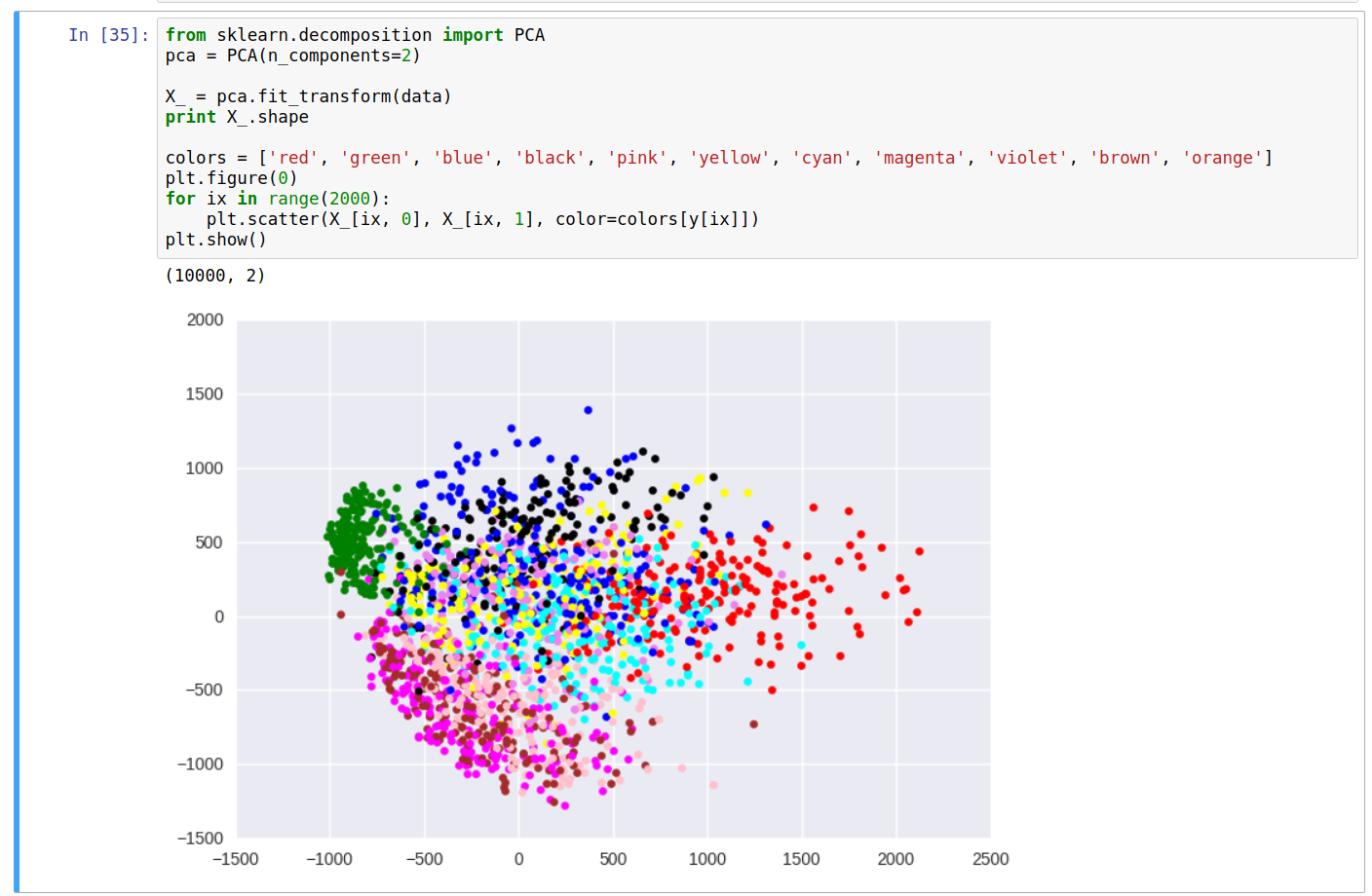From the scatter plot, we can do some simple analysis and see some relationship between the color of points (labels) and their location on the plot. For instance, the green cluster (representing the label 1) is formed clearly distinct from others, while the clusters for colors brown and pink (for digits 4 and 9) are somewhat in the same region, etc.

Although the explained variance with 2 dimensions was roughly 23%, we still can derive some meaningful information about the data. Having more number of dimensions will make it easier to process and analyse the data as compared to the original data distribution.

Also, applying PCA would make it easier to use the data in models such as the Naive Bayes, where the core assumption is that the columns are independent of each other.

Note: If we want to keep the physical meaning of the columns in the dataset intact, using PCA would be a bad idea since the transformed columns are linear combinations of the original columns. Hence, the new columns would lose their original meaning.

Also, dimension reduction is useful only if the eigenvalues vary significantly for any data distribution. For eigenvalues in similar ranges, each column will have similar contribution towards the variation in data, hence removing them would cause greater loss.

]]>
Object Detection with R-CNN Family2017-12-05T09:00:00+00:002017-12-05T09:00:00+00:00https://shubham1810.github.io/blog/2017/objectdetectionConvolution Neural Networks (CNNs) are widely used, majorly for the purpose of image classification (classifying an object in an image into one of the given categories) and have shown to perform very well on huge datasets (for example, the ImageNet challenge [link]). Even with the huge success of CNNs in classification, the task of actually understanding an image still remains a challenge. One such task that corresponds to image understanding is object detection, wherein the task is to detect objects in an image and specify where these objects appear in the image (using a bounding box or masking etc.).

Several algorithms have been proposed to solve the task of object detection, and one such class of methods to be discussed in this post is the R-CNN family of algorithms (R-CNN [], fast R-CNN [], faster R-CNN [], Mask R-CNN []).

## R-CNN

R-CNN, or Regions with CNN features, is a method for object detection proposed in 2014

]]>
Markov Chains2017-10-14T09:00:00+00:002017-10-14T09:00:00+00:00https://shubham1810.github.io/blog/2017/mcMarkov chains are memoryless mathematical process (or a sequence) which jump from one state to another, following the rules of the Markov property. A state can be thought of as a situation/event or a set of values. One example to demonstrate a markov chain can be weather conditions; Sunny and Rainy being two weather conditions (states), one such sample of a sequence of events can be as follows:

The Markov chain follows the shifts or transitions based on a Transition Probability Matrix, $$T$$, which contain information about how probable it is to visit state $$j$$ when the current state is $$i$$, for all possible states of the system (called the state space, $$S$$). The Markov property states that the conditional probability distribution of the future states depends only on the present state, not the sequence of previous states. Mathematically, assume $$X$$ is a sequence of states $$x_i \in S$$, then $$X = x_n, x_{n-1}, ..., x_0$$ is a Markov sequence iff:

$\mathbb{P}(X_n = x_n | X_{n-1} = x_{n-1}, ..., X_0 = x_0) = \mathbb{P}(X_n = x_n | X_{n-1} = x_{n-1})$

Where the probability of transition is taken from $$T$$, i.e.

$\mathbb{P}(X_n = j | X_{n-1} = i) = T_{ij}; T = \begin{bmatrix} p_{11} & p_{12} & p_{13} & \dots & p_{1m} \\ p_{21} & p_{22} & p_{23} & \dots & p_{2m} \\ \vdots & \vdots & \vdots & \ddots & \vdots \\ p_{m1} & p_{m2} & p_{m3} & \dots & p_{mm} \end{bmatrix}$

It is because of the markov property that the markov chain is called a memoryless process since there is no requirement to store the past states in the memory. The system jumps from one state to another following the probability distribution given by the transition probability matrix $$T$$. An excellent interactive example of a markov chain can be found here.

Also, since markov chains predict the probability of going from a state $$i$$ to state $$j$$ ($$i, j \in S$$) in one step, they can also be used to predict the probability of going from state $$i$$ to state $$j$$ in some $$k$$ number of steps. The probability of going from $$i$$ to $$j$$ in 2 steps (reaching an intermediate state $$p$$ in between) $$i \to p \to j$$ is:

$\mathbb{P}(X_n = j | X_{n-1} = p) . \mathbb{P}(X_{n-1} = p | X_{n-2} = i) = T_{ip} . T_{pj}$

which is essentially the element at position ($$i, j$$) in a matrix $$A = T^2$$. In general, this probability for $$k$$ steps can be computed from $$T_{ij}^{k}$$.

Few popular applications of Markov chains include Google PageRank, Autocomplete/typing word prediction, Generating sequences of text (for sentences) or pixels (for images) etc.

TODO: Add code + example for text generation using markov chains.

]]>
Evolutionary Algorithms I: Differential Evolution2017-06-15T09:10:00+00:002017-06-15T09:10:00+00:00https://shubham1810.github.io/blog/2017/deEvolutionary Algorithms are classified under a family of algorithms for global optimization by biological evolution, and are based on meta-heuristic search approaches. The possible solutions usually span a n-dimensional vector space over the problem domain and we simulate several population particles to reach a global optimum.

An optimization problem, in a basic form, consists of solving the task of maximizing or minimizing a real function by choosing values from a pool of possible solution elements (vectors) according to procedural instructions provided for the algorithm. Evolutionary approaches usually follow a specific strategy with differenet variations to select candidate elements from population set and apply crossover and/or mutations to modify the elements while trying to improve the quality of modified elements.

These algorithms can be applied to several interesting applications as well, and have been shown to perform very well in optimizing NP-hard problems as well, including the Travelling Salesman Problem, Job-Shop Scheduling, Graph coloring while also having applicaitons in domains such as Signals and Systems, Mechanical Engineering, and solving mathematical optimization problems.

One such algorithm belonging to the family of Evolutionary Algorithms is Differential Evolution (DE) algorithm. In this post, we shall be discussing about a few properties of the Diferential Evolution algorithm while implementing it in Python (github link) for optimizing a few test functions.

## Differential Evolution

DE approaches an optimization problem iteratively trying to improve a set of candidate solutions for a given measure of quality (cost function). These set of algorithms fall under meta-heuristics since they make few or no assumptions about the problem being optimized and can search very large spaces of possible solution elements. The algorithm involves maintaining a population of candidate solutions subjected to iterations of recombination, evaluation and selection. The creation of new candidate solution requires the application of a linear operation on selected elements using a parameter $$F$$ called differential weight from population to generate a vector element and then randomly applying crossover based on the parameter Crossover Probability. $$CR$$.

The algorithm follows the steps listed down:

1. Initialize a set of agents/elements $$x$$ with random positions in the search space for population size $$P$$.
2. Until a termination criterion is met (number of iterations or required optimality), repeat the following for each agent $$x_i$$:
• Pick three agents $$a, b$$, and $$c$$ from the population at random (distnct).
• Pick a random index $$R \in \{1,...,n\}$$ ($$n$$ is the dimensionality of the problem)
• Compute a temporary vector $$y$$ as following:

$y = a + F (b-c)$
• Now, for each $$j \in \{1,...,n\}$$, pick a uniformly distributed number $$r_i \equiv U(0, 1)$$.
• If $$r_i \lt CR$$ or $$i=R$$, then
• set $$x_{I, j} = y_{j}$$
• Otherwise, $$x_{I, j} = x_{i, j}$$

• if $$f(x_{I}) \lt f(x_i)$$, ($$f$$ is the cost function for minimization), then
• replace $$x_i$$ with $$x_i$$.
• otherwise, $$x_i$$ remains unchanged.
3. Pick the agent from the population that has the highest fitness or lowest cost function value as the solution.

## Implementing the Algorithm

The directory structure for the code follows the design as given below:

Where, differential_evolution.py is the main file we’ll run for execution of the algorithm. The helpers directory consists of helper classes and functions for several operations such as handling the point objects and vector operations related to candidate elements (point.py), methods for handling the collection of all such points and building the population (collection.py), test functions to be used objective/cost functions for testing the efficiency of the algorithm (test_functions.py).

### Building The Point Class

Here, we’re initializing the Point class with dim which is the dimension size of the vector, lower_limit and upper_limit specify the domain of each co-ordinate of the vector. self.z is the objective function value of the point, associated with each instance to make it wasy for ranking them based on their objective function value. The evaluate_point function runs the objective function for the given point on the test function. The Point class creates instance of vector objects signifying each individual in the population. The collection of individuals is defined in the Population class.

### The Population Class

The Population class contain the set of point class instances acting a individuals in the population. The individuals are stored in self.points list. The parameters of the class are num_points, containing information about the population size, dim, upper_limit and lower_limit as discussed above. As an optional parameter, init_generate controls the generation of the initial population and objective referes to an object of the Function class and is the objective function (discussed in the next section). If set to False, the initial population will be empty and the elements will need to added through the main procedure of the algorithm. The get_average_objectve function returns the mean evaluated objective value of the population.

### The Objective Functions

The test_functions.py contains the implementation of the Function class, which creates an objecctive function object. The parameters to the constructor is func which can either be a string or a function. If None, it’ll store the function sphere in self.func, else it shall check for string value. For a string, it will assign the function with the same name implemented in the class (stored under the dictionary self.objectives). For a function, this assumes that the function accepts a numpy ndarray as an input and returns a scalar quantity as the objective function value.

The Objective functions implemented by default currently include sphere, ackley, rosenbrock, and rastrigin functions. A list of optomization test functions can be found here. These are all defined in a multi-dimmensional vector space and exhibit either unimodal or multi-modal properties. For example, the sphere function is a unimodal convex function, while the rastrigin function is a multi-modal non-convex function. The representation of the rastrigin function in a 3-D space is shown (the vertical axis is the value of the objective function):### The Differential Evolution Class

Here, in the DifferentialEvolution class, the initializing parameters are:

1. num_iteration controlling the number of generations/iterations the optimization loop runs. Acts as the stopping criterion.
2. CR and F are the Crossover Probability and the Differential Weight as defined in the algorithm.
3. dim is the number of dimensions of the individial vectors (Size of the vector space, $$x \in R^n$$; $$x$$ is an individual vector).
4. population_size is passed to the Population class and the population object is stored in self.population.
5. print_status is a boolean value used for verbosity (prints the best objective function value at each iteration).
6. func accepts either the function name or the actual function and is used to create the self.func object, which is an instance of the Function class.
7. self.iteration keeps tracck of the current iteration/generation.

There are essentially two member functions, self.iterate and self.simulate. The self.iterate function runs oone iteration of the Differential Evolution procedure, by applying the transformation operation and crossover on each individual in the population, and the self.simulate function calls the iterate function until the stopping criteria is met, and then prints the best value for the objective function.

### Demo

Now that we have an implementation for all the required classes for the Differential Evolution algorithm, we can write a small script to test everything out and see the results.

This script initializes the variables number_of_runs, val, and print_time. number_of_runs is used to initiate several runs of the algorithm, and finally the average outcome of the optimized objective function is returned after those runs. val stores the optimized objective function value for each run and is later used to compute the average. print_time is a boolean which controls if the computation time should be printed for each run or not.

The output for the above code, i.e. using the differential evolution algorithm to optimize the sphere test function, on 50 dimensions (50-D vector space), running for 200 iterations for each runs produces the following output:

The plot for objective function value against the iterations for the sphere test function in 50D and the Rastrigin test function in 50D are shown below:The code is available in a github repository here.

]]>